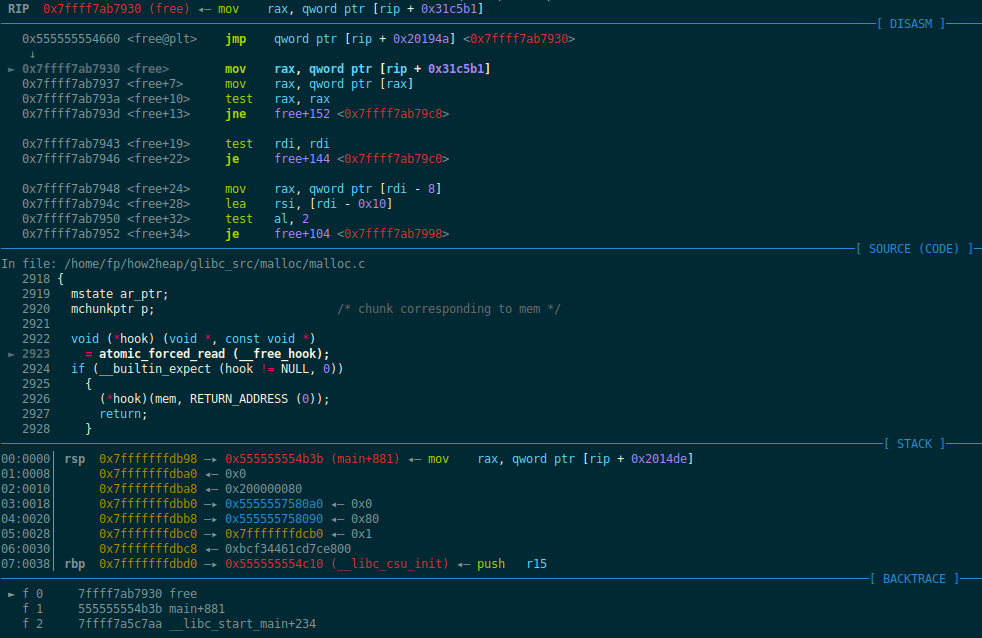## 如何进行源码调试

### 首先使用how2heap提供的脚本build出实验需要的2.25版本的ld和libc，如下：

``````\$ ./glibc_build.sh -h
\$ ./glibc_build.sh 2.25 8
``````

### 接下来修改待调试文件的ld,有两种方法，可以自由选择：

``````# 首先要sudo apt install patchelf，以下两种方法实际上都是用了这个工具。
# 1. 使用现成的glibc_run.sh，脚本中含有patch待调试程序的功能。
\$ ./glibc_run.sh 2.25 [exe]
# 2. 直接使用patchelf
\$ patchelf --set-interpreter [path_to_ld] [exe]
```````ni`了几步之后进入了_int_free函数，这也就是我们分析的重点。``````#define unlink(AV, P, BK, FD) {                                            \
FD = P->fd;                                                               \
BK = P->bk;                                                               \
if (__builtin_expect (FD->bk != P || BK->fd != P, 0))                     \
malloc_printerr (check_action, "corrupted double-linked list", P, AV);  \
else {                                                                    \
FD->bk = BK;                                                          \
BK->fd = FD;                                                          \
if (!in_smallbin_range (P->size)                                      \
&& __builtin_expect (P->fd_nextsize != NULL, 0)) {                \
// 与本次实验无关                                                    \
......                                                            \
}                                                                   \
}                                                                       \
}
``````

``````static void
_int_free (mstate av, mchunkptr p, int have_lock)
{
......
``````

``````static struct malloc_state main_arena =
{
.mutex = _LIBC_LOCK_INITIALIZER,
.next = &main_arena,
};
......
mstate ar_ptr = &main_arena;
``````

• 前向合并只有检测到下一个chunk不是当前分配区的topchunk并且是未使用状态才会触发，合并结果是将后chunk合并入前chunk，新的chunk开始于前chunk，大小为两个chunk之和。若下一个chunk就是topchunk，则直接将当前chunk变为topchunk。``````0x7ffff7ab4710 <_int_free+416>    test   byte ptr [rbx + 8], 1
=> rbx= p =0x555555758090

0x7ffff7ab4716 <_int_free+422>    mov    rax, qword ptr [rbx]
=> rax=0x80

0x7ffff7ab4719 <_int_free+425>    sub    rbx, rax
=> rbx -= 0x80
=> rbx= p =0x555555758010  <-------------- fake mchunkptr

=> r12= size =size+prevsize=r12+rax=0x90+0x80=0x110
````````````-------------------unlink-------------------
0x7ffff7ab471f <_int_free+431>    mov    rax, qword ptr [rbx + 0x10]
=> rax= FD =p->fd=0x555555756018

0x7ffff7ab4723 <_int_free+435>    mov    rdx, qword ptr [rbx + 0x18]
=> rdx= BK =p->bk=0x555555756020

0x7ffff7ab4727 <_int_free+439>    cmp    qword ptr [rax + 0x18], rbx
=> test FD->bk==p

0x7ffff7ab4731 <_int_free+449>    cmp    qword ptr [rdx + 0x10], rbx
=> test BK->fd==p

0x7ffff7ab473b <_int_free+459>    cmp    qword ptr [rbx + 8], 0x3ff
=> test p->size>small_bin_range

0x7ffff7ab4743 <_int_free+467>    mov    qword ptr [rax + 0x18], rdx
=> FD->bk = BK
=> qword ptr [0x555555756030] = 0x555555756020

0x7ffff7ab4747 <_int_free+471>    mov    qword ptr [rdx + 0x10], rax
=> BK->fd = FD
=> qword ptr [0x555555756030] = 0x555555756018
=> qword ptr [&chunk0_ptr] = ((char *)&chunk0_ptr)-0x18

p->size in small_bin_range, so unlink finish.
-----------------------------------------------
``````

Tags:

Categories:

Updated: